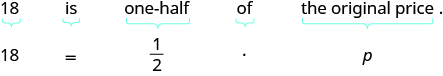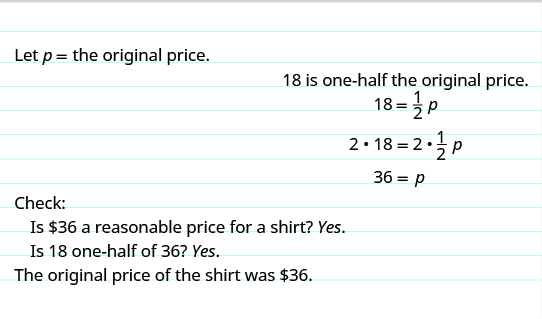## Algebraic Problem Solving Strategies

### Learning outcome

• Use a problem-solving strategy to set up and solve word problems

The world is full of word problems. How much money do I need to fill the car with gas? How much should I tip the server at a restaurant? How many socks should I pack for vacation? How big a turkey do I need to buy for Thanksgiving dinner, and what time do I need to put it in the oven? If my sister and I buy our mother a present, how much will each of us pay?Now that we can solve equations, we are ready to apply our new skills to word problems.

Previously, you translated word phrases into algebraic equations using some basic mathematical vocabulary and symbols. Since then you’ve increased your math vocabulary as you learned about more algebraic procedures. You’ve also solved some word problems applying math to everyday situations. This method works as long as the situation is familiar to you and the math is not too complicated.

Now we’ll develop a strategy you can use to solve any word problem. This strategy will help you become successful with word problems. We’ll demonstrate the strategy as we solve the following problem.

### Example

Pete bought a shirt on sale for \$$18$, which is one-half the original price. What was the original price of the shirt?

Solution:
Step 1. Read the problem. Make sure you understand all the words and ideas. You may need to read the problem two or more times. If there are words you don’t understand, look them up in a dictionary or on the Internet.

• In this problem, do you understand what is being discussed? Do you understand every word?

Step 2. Identify what you are looking for. It’s hard to find something if you are not sure what it is! Read the problem again and look for words that tell you what you are looking for!

• In this problem, the words “what was the original price of the shirt” tell you what you are looking for: the original price of the shirt.

Step 3. Name what you are looking for. Choose a variable to represent that quantity. You can use any letter for the variable, but it may help to choose one that helps you remember what it represents.

• Let $p=$ the original price of the shirt

Step 4. Translate into an equation. It may help to first restate the problem in one sentence, with all the important information. Then translate the sentence into an equation.Step 5. Solve the equation using good algebra techniques. Even if you know the answer right away, using algebra will better prepare you to solve problems that do not have obvious answers.

 Write the equation. $18=\Large\frac{1}{2}p$ Multiply both sides by 2. $\color{red}{2}\cdot18=\color{red}{2}\cdot\Large\frac{1}{2}\normalsize p$ Simplify. $36=p$

Step 6. Check the answer in the problem and make sure it makes sense.

• We found that $p=36$, which means the original price was $\text{\36}$. Does $\text{\36}$ make sense in the problem? Yes, because $18$ is one-half of $36$, and the shirt was on sale at half the original price.

Step 7. Answer the question with a complete sentence.

• The problem asked “What was the original price of the shirt?” The answer to the question is: “The original price of the shirt was $\text{\36}$.”

If this were a homework exercise, our work might look like this:### Try it

We list the steps we took to solve the previous example.

### Problem-Solving Strategy

1. Read the word problem. Make sure you understand all the words and ideas. You may need to read the problem two or more times. If there are words you don’t understand, look them up in a dictionary or on the internet.
2. Identify what you are looking for.
3. Name what you are looking for. Choose a variable to represent that quantity.
4. Translate into an equation. It may be helpful to first restate the problem in one sentence before translating.
5. Solve the equation using good algebra techniques.
6. Check the answer in the problem. Make sure it makes sense.
7. Answer the question with a complete sentence.

Let’s use this approach with another example.

### Example

Yash brought apples and bananas to a picnic. The number of apples was three more than twice the number of bananas. Yash brought $11$ apples to the picnic. How many bananas did he bring?

### Try it

In the next example, we will apply our Problem-Solving Strategy to applications of percent.

### example

Nga’s car insurance premium increased by $\text{\60}$, which was $\text{8%}$ of the original cost. What was the original cost of the premium?

### Try it

Now we will translate and solve number problems. In number problems, you are given some clues about one or more numbers, and you use these clues to build an equation. Number problems don’t usually arise on an everyday basis, but they provide a good introduction to practicing the Problem-Solving Strategy. Remember to look for clue words such as difference, of, and and.

### Example

The difference of a number and six is $13$. Find the number.

Solution:

 Step 1. Read the problem. Do you understand all the words? Step 2. Identify what you are looking for. the number Step 3. Name. Choose a variable to represent the number. Let $n=\text{the number}$ Step 4. Translate. Restate as one sentence. Translate into an equation. $n-6\enspace\Rightarrow$ The difference of a number and 6 $=\enspace\Rightarrow$ is $13\enspace\Rightarrow$ thirteen $n-6=13$ Step 5. Solve the equation. Add 6 to both sides. Simplify. $n-6=13$ $n-6\color{red}{+6}=13\color{red}{+6}$ $n=19$ Step 6. Check: The difference of $19$ and $6$ is $13$. It checks. Step 7. Answer the question. The number is $19$.

### example

The sum of twice a number and seven is $15$. Find the number.

### try it

Watch the following video to see another example of how to solve a number problem.

## Contribute!

Did you have an idea for improving this content? We’d love your input.